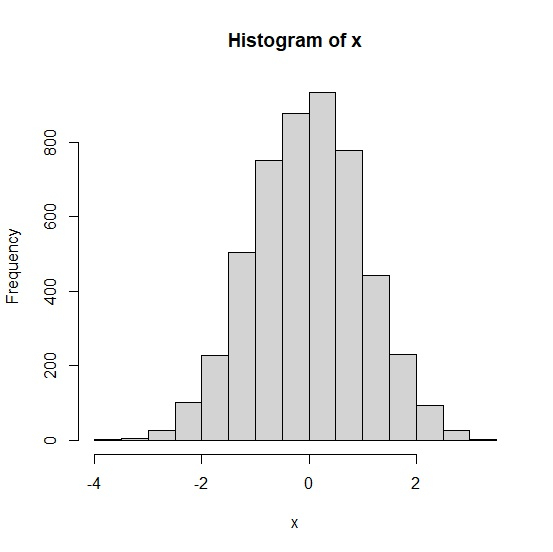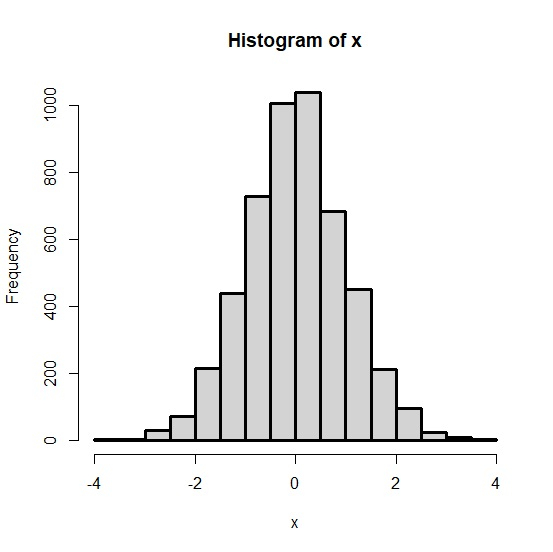# How to increase the thickness of histogram lines in base R?

To increase the thickness of histogram lines in base R, we would need to use par function by defining the thickness size of the line. If we want to do so then line thickness must be defined first before creating the histogram. An example of line size could be line<-par(lwd=3).

## Example

x<-rnorm(5000)
hist(x)

## Output## Example

line<-par(lwd=3)
hist(x)

## Output